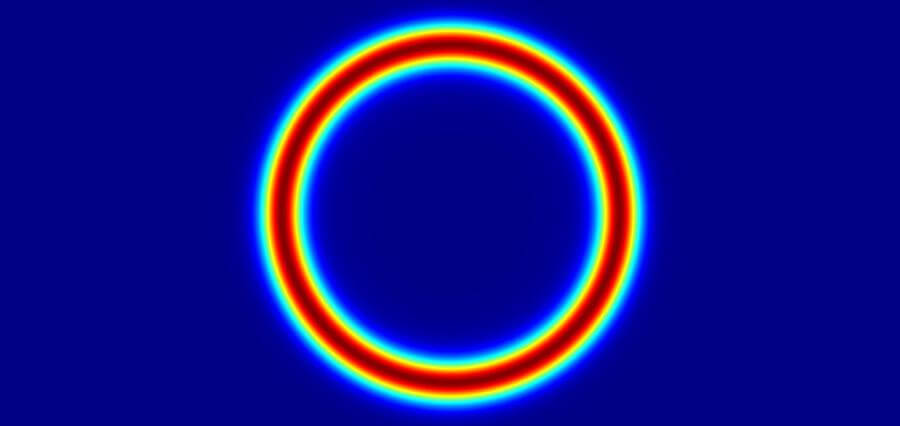# Diffractive Optics

Diffractive Optics can be regarded as one important field of optical engineering.  It is a discipline for designing optical systems and components by taking full advantage of the wave properties of light. This is in contrast with the more common optics design discipline, geometrical or ray optics, in which a beam of light is treated by a pencil of rays with no wave interference occurring whatsoever. In many situations of interest, geometrical optics is very accurate and efficient. In fact, the most common optical components, lenses and mirrors, for example, are always designed following the principles of diffractive optics.

Despite the evident huge success of geometrical optics, diffractive optics offers many advantages and possibilities that geometrical optics cannot provide nor explain. By harnessing the ability to produce wave interference on a single beam, diffractive optics can be used to design optical components that can be very thin but at the same time very effective in performing rather complex transformations on a given input beam.

In geometrical optics, the surface of a component, such as a face on a lens, is smooth and continuous. In contrast, the surface of a component designed using diffractive optics is a discretised array of small modulating elements. Thus, when a beam traverses such a component the different elements, which can be referred to simply as pixels, modulate the wavefront of the input beam differently at each point.  The overall wavefront then changes drastically and then, as a result of diffraction, a new beam output profile emerges after some propagation distance.  Diffraction is then the interference phenomenon that occurs among many waves at the same time. This is what is actually happening when the beam traverses the modulating array. The beam is kind of being portioned into smaller beams that then interfere among themselves.

Diffractive Optics design relies partly on ray tracing, but at the core of diffractive optics, there is the solution to the diffraction integral, which is obtained using the mathematical formalism derived from the wave theory of light. In its most primaeval form, such mathematical relations are not easy to handle. Fortunately, in many scenarios of practical interest, it can be reduced to a more tractable formula that can be computed numerically using algorithms already developed and that are currently used in modern signal processing engines. Namely, the diffraction integral can be reduced to a simple spatial Fourier transform that is scaled according to the spatial dimensions involved.